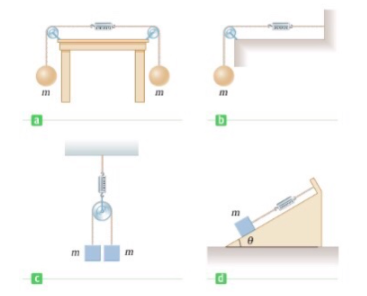# Problem: The systems shown below are in equilibrium. If the spring scales are calibrated in newtons, what do they read? Ignore the masses of the pulleys and strings and assume the pulleys and the incline are frictionless. (Let m = 2.46 kg and θ = 29.0°)scale in (a) = _ Nscale in (b) = _ Nscale in (c) = _ Nscale in (d) = _ N

###### FREE Expert Solution

In this problem, we have a set of forces (weights) and tensions.

We'll use Newton's second law and free body diagrams.

Newton's second law:

$\overline{){\mathbf{\Sigma }}{\mathbf{F}}{\mathbf{=}}{\mathbf{m}}{\mathbf{a}}}$

84% (244 ratings)###### Problem Details

The systems shown below are in equilibrium. If the spring scales are calibrated in newtons, what do they read? Ignore the masses of the pulleys and strings and assume the pulleys and the incline are frictionless. (Let m = 2.46 kg and θ = 29.0°)
scale in (a) = _ N
scale in (b) = _ N
scale in (c) = _ N
scale in (d) = _ N